﻿ A New Topology to Improve Performance of SAPF Using ZVSPublications are Open
Access in this journal
Article Versions
Export Article
• Normal Style
• MLA Style
• APA Style
• Chicago Style
Research Article
Open Access Peer-reviewed

### A New Topology to Improve Performance of SAPF Using ZVS

Hassan Shadkam Anvar, Mojtaba Bahreini Pour, Ali Barmakh
International Transaction of Electrical and Computer Engineers System. 2019, 6(1), 8-18. DOI: 10.12691/iteces-6-1-2
Received December 10, 2018; Revised January 12, 2019; Accepted February 16, 2019

### Abstract

This paper presents new topology using soft switching to improve performance of shunt active power filter (SAPF). The proposed inverter can be used without employing Snubber capacitor under Zero voltage switching and high frequency conditions. To create ZVS conditions for the switches in this converter, an auxiliary circuit is used that includes two IGBT and LC resonant circuit. Soft switching causes the effective improvement of active filter performance and lowers stress voltage level effectively and the strokes of switch voltage as well as decrease of EMI emission reduction. In addition, the new topology will eliminate the problem of reverse recovery of diode of components body, and in addition, voltage stress on the PI controller, will greatly reduce used in power active filter. Today, with advances in power electronic technology and increasing use of power converters and other non-linear loads in the harmonics industry existing in the power system have largely increased that from the adverse effects of harmonics, the losses increase and incorrect performance of power lines equipment and interferential issues of waves can be named in telecommunication lines. Power active filters are used to eliminate harmonics of current and voltage. Numerous method of performance that use an active auxiliary circuit to help the main switch of a PWM modulated converter switch on with zero voltage switching have been proposed. These methods reduce switching losses in the main power switch, reduce reverse-recovery losses in the main power diode, and reduce EMI in the converter. Although closely all previously proposed auxiliary circuits include only a single active switch because of cost, but this paper used Two-switch auxiliary circuits for ZCS-PWM converters are usually used in high current applications..By the way to simulate this topology has been used of MATLAB and CADENCE softwares.

### 1. Introduction

In recent years, the increase of non-linear loads, have caused undesirable phenomenon in performance of power systems. These loads have non-linear impedance to the ac current and their current waveform gets distortion 1 relative to the sinus pure waveform of base frequency that is desirable to the grid. On the other hand, due to the limitations considered by different standards especially for the industrial consumers, the decrease of harmonic problems resulting from load is posed as a necessity. Therefore, different types of Compensation instruments have been introduced for this purpose. Passive filters have been proposed to eliminate the current harmonics and improve the power coefficient. Although, this filter class enjoys less simplicity and cost, but due to the line impedance effect and the likelihood of erupting the resonance problems of inability to eliminate several harmonic components enjoy less acceptance. One of these compensation instruments is passive capacitive-inductive (LC) filter; these filters cause the improvement of coefficient quality by simple and cheap designing; however, problems such as large size, unwanted oscillations has become the reason to reduce the use of these topologies. 2, 3.

The power active filter is one the most efficient compensating instruments that enjoys large application in the distribution systems, which have the ability to eliminate all undesired harmonics of power coefficient improvement. During few recent decades, different structures of active filters have been presented and offered 4, 5, 6, 7. Nowadays, active filters are used more due to their better performance as compared to passive filters, more regulating ability, less size and weight to eliminate harmonics and improve the power coefficient. The active filters based on the inverter types, topology, numbers of phases, and as well as the controlling strategy are divided into different classes 8. The working base of active filter is in this way: the harmonics equivalent to the harmonics of line current, but to its opposite phase, is injected to the line to compensate the harmonics of line current 9.

The use of high-efficiency switching converters is preferred over voltage regulators with low efficiency. In many converters an auxiliary circuit is used 10, 11 to provide soft switching conditions. This employed auxiliary circuit has one or more extra switches that are the new kind of topology of soft switching implementation. In reference 12 a number of methods have been presented to decrease the switch voltage stress. Although, the use of Snubber circuit in inverter switching reduces the EMI, but may increase losses in the circuit and as a result would reduce efficiency 12, 13. During recent years, soft switching methods have been used to remove this complication and the performance of inverter for higher frequencies; in addition, the high withstand voltage switch should be used that usually the conduction resistance .i.e. it's RDS (on) gets more and causes the increase of conductive losses, switch price and efficiency decrease. On the other hand, the possibility of optimal designing of magnetic elements is not available due to the control of varied frequency. The active clamp technique is also used in some circuits 14, 15. The suggestive soft switch used in the active filter not only would not cause the harmonic decrease of current source, but will decrease the voltage stress intensively at the input of PI controller block in active power filter.

### 2. Principles and Practice of Active Filter with Soft Switch

Nowadays, the use of non-linear loads are growing strongly that would be a reason to create harmonic current sources is shown in Figure 1. Therefore, to reduce harmonics created in the current source is the cheapest and most reliable way to use active filter that the soft switch can be used to improve its performance in active filter control unit.

Using ZVS-PWM in active filter control circuit associates abundant privileges:

1. Reduce voltage stress on the active filter components that the PI controller block can be referred for and an error signal enters in it.

2. Increasing the efficiency of parallel active filter.

3. Use of appropriate PWM circuit.

4. Decrease of Vdc overshoot voltage at the input of PI controller.

5. Time reducing to reach an output voltage to an acceptable value.

2.1. Parallel Active Filter Topology

1. Achieving compensated current proportional to voltage.

2. Achieving compensated current with the least effective amount of power that have the ability to distribute to the main load current.

There are two types of control strategy for active p-q filters: the theory of instantaneous power and the theory of time-domain, the time domain are only used for three-phase circuits and Fourier analysis in the frequency domain. The time-domain methods are mostly used to achieve faster with less computations in comparison with the methods used for frequency domain 16. Today, in the majority of active filters, the instantaneous reactive power theory is used as a control strategy.

• Figure 1. Use of soft switch in the active filter power circuit
2.2. Instantaneous Powers of P-Q Theory

In the p-q theory of three phase systems, three powers by using phase voltage and the instantaneous line currents are introduced in α β 0 axes:

Zero sequence power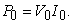(1)

Real power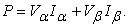(2)

Imaginary power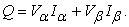(3)

The real power has been calculated using the voltages and currents of ac direction that is equal to a power that is obtained as a result of dc current multiplication with dc voltage coefficient, but the imaginary power is only calculated in ac direction. In three wired three phases system there is no zero sequence current components; hence I0 is equal to zero, it indicates that only the real and imaginary powers exist in α β axis.

2.2.1. Current Controller Designing to Follow Reference Current

Parallel active filter can also provide harmonic damping across the transmission line's length so that to prevent the harmonics dissemination resulted from the resonance between series inductance and parallel capacitor, correcting the power coefficient.

1. Calculate the instantaneous power (Clark Conversion).

2. Choosing compensating power (Instantaneous Power).

3. Dc voltage regulator (PI Controller).

4. Reference current computation (Clark Reverse Conversion).

5. Flow or current control.

2.2.1.1. Clark Conversion

Αβ0 conversion is known as Clark conversion, that could convert three-phase instantaneous voltages in abc .i.e. Va, Vb, Vc to instantaneous voltages in αβ0 axis, Va ، Vb ، Vc. Recently an instantaneous reactive theory has been presented in a-b-c sequence in 14 that has no need of coordinate conversion is shown in Figure 2. And In equation (7, 5) has been shown how Calculation of Voltage and reference current by Clark conversion: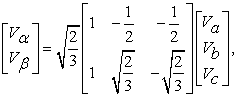(4)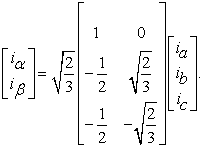(5)

In Clark conversion the Instantaneous values of the phase voltages and line currents related to abc Stationary axis are transferred to the Stationary axes of αβ and inversely.

• Figure 2. Control block for constant instantaneous power

2.2.1.2. Choosing Compensating Power (Instantaneous Power)

Instantaneous power block calculates the instantaneous power of nonlinear load and determines the behavior of active filter; in simple expression it selects the real and imaginary nonlinear load that should be compensated by a parallel active filter. Also on the base of p-q theory, there exist only the real and imaginary powers and the zero sequence power is always zero.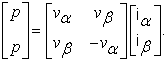(6)

For the instantaneous power constant from source, the parallel active filter should be nearby to the non-linear load carrying location; to compensate the real oscillating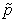power. In equation (7) powers finding has been shown through voltages and currents. The real and imaginary current power can be decomposed in power systems to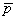andcomponents 16, 17, 18.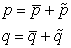(7)

Medium oscillations powers + oscillating powers= instantaneous dc components that is related to the main component of active current.= instantaneous ac components of p that is related to that group of harmonic currents that is produced by the active instantaneous power component.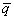= is the dc component of instantaneous power and related to the reactive power produced by the main components of current and voltage.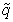= is the ac component of instantaneous power of q and related to that class or group of harmonic currents that is produced by reactive component of instantaneous power. In addition,andexpress the oscillating and intermediate components of p. whereas,andexpress the imaginary part of q. In this article, the parallel active filter was used to compensate the constant power that this method provides the optimal compensating ability from the perspective of energy supply for non-sinusoidal and unbalanced voltages is shown in Figure 3.

• Figure 3. Optimized power supply obtained from parallel power filter compensation

2.2.1.3. Dc Voltage Regulator (PI Controller)

In practical implementation of a dc voltage regulator a controller can be used that in fact by this practice the quantitative amount of average real power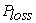will be pulled uninterruptedly from the power system to satisfy the imaginary switching losses of PWM inverter. In addition, In addition, the smaller the dc capacitance, the greater cutoff frequency should be chosen for Butterworth filter for parallel active filter controller. This block by a PI controller, achieves the reference voltage from proportion between the determined voltage and capacitor voltage. By setting the PI controller share that has an important role in dc voltage regulation, the PI controller system response can be increased to input. In fact,is not only the losses compensator, but also prevents dc voltage changes from thelow frequency components.is the loss obtained from passive elements in active filter that causes voltage drop of dc capacitor. Therefore, thesignal is very important to create energy balance in active filter. The diagram block of PI controller is shown in Figure 4.

• Figure 4. PI controller schematic

As well as, the PI controller or the very proportional block makes the Integrator dc capacitor voltage of same phase with supply voltage that improves the power factor of the current supply unit.

2.2.1.4. Reference Current Computation (Clark Reverse Conversion)

The reference currents of i, i active filter are obtained by converting the signals of compensating current of i*Ca, i*Cb, i*Cc to coordinates. In equation (8), the equation of Clark inverse conversion is shown and in addition, Figure 5 of diagram block related to reference current calculation shows the instantaneous reactive power in the theory.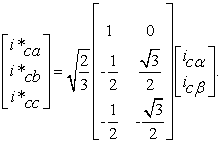(8)

2.2.1.5. Power Controller Circuit And Compensator Controller

1. Power circuit (PWM inverter).

2. Compensator controller circuit (Hysteresis Controller).

• Figure 5. Clark's reverse conversion block
• Figure 6. PWM inverter

Power circuit of parallel active filter includes three-phase voltage inverter that is composed of six IGBT pieces and parallel diodes. The PWM inverter shown in Figure 6 should accurately provide the real oscillating power of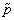that is removed from the power supply. Therefore, the PWM inverter must also have the ability of energy production and absorption, of course, with zero amounts. As a result, the PWM inverter must be connected to an energy storing system (capacitor) that is mounted to a power circuit. The PWM inverter must have a high switching frequency circa 10 times equivalent to the highest harmonic frequency. IGBT parts are used in the PWM inverters that behave like a controlled current supply and to avoid the high di/dt, the connection should be made to the main power system through inductor-series. The PWM inverter frequency should not be equal to the stimulus resonance frequency. The Hysteresis Controller is a PWM method that forces the inverter to act as voltage supply and in addition, the PWM controller that is used in this paper is a PWM sinusoidal type controller based on carrier-based sinusoidal PWM control 16. This type of switching is based on the comparison of sinusoidal reference signal with triangular wave and on the other hand it is the switching in the main frequency that forces the inverter to produce a square wave output that has main component or the amplitude that is direct and appropriate to the dc voltage through voltage supply. Therefore, the output frequency and phase can be changed abruptly by instantaneous regulation of switching, whereas, their amplitude is changed when the dc voltage is also changed is shown in Figure 6.

• Figure 7. Hysteresis Controller inverter

All active filters use voltage supply PWM inverter in practical applications 19.

### 3. Soft Switch Topology

The ZVS-PWM inverter can widely be used in power systems and industrial inverters, in addition, this suggestive inverter is able to be employed in high power and frequency. As well as, their performance frequency must be increased to reduce the size and weight of these inverters; however, the frequency increase also causes the switching loss increase, appropriate performance can be predicted by presenting this technique. The overall structure of the proposed inverter is composed of a relatively large inductor at the entrance, one main switch as well as, two auxiliary switches which are mainly used in the high current circuits 20.

The proposed ZVS-BOOST inverter that is seen in Figure 8 consists of 1 main switch with 2 Saux1 and Saux2 ، auxiliary switches, 4 d1, d2, d3, d4 diodes and 1 Cr and Lr resonance tank circuit.

• Figure 8. Proposed ZVS with auxiliary switch

Initial functioning principle of this inverter is as follows: the Saux1 auxiliary switch turns on just before turning on of the main switch, in the meantime the current flows in the circuit through diode D4 that has been flown inversely in the circuit and the Cout capacitor has been fully discharged while its voltage value reaches zero. The main switch has the ability to turn on with ZVS, just at the time of full discharge of Cout capacitor. Because of the presence of Cr and Lr resonance circuit related to auxiliary circuit current, then (Saux1, Saux2) is almost zero that the Saux1 switch will be turned on through ZCS. These conditions also provide ZVS switching at zero voltage for the main switches as well as for the auxiliary circuit switches. On the other hand, the additional chip is not necessary for auxiliary circuit switches and as the control command for the using IGBT as the switch is of PWM type; hence, a very simple and cheap controller can be proposed and presented for it. The privilege of this proposed converter has been made suitable for high power applications; in addition, each switch also has a diode in series with it to prevent the inverse current flow. The major use of soft switch in active filter controller block is to decrease the voltage stress the PI controller and increase the Speed of error detection accuracy in it.

3.1. Resonant Performance of Proposed Converter

The proposed converter in boost state has 10 different performance conditions in one switching course of (ts = t0 ~ t9), as shown in Figure 9. it is supposed that all switches will turn off before the time t0.

• Figure 9. 10 State’s proposed soft switch inverter
• Figure 10. Switching waveforms

3.1.1. The First Condition of (A) Figure

In this mode .i.e. the condition that is t<t0, all inverter switches are off and the current will arrive through D4 to output.

3.1.2. The Second Condition of (B) Figure

In this mode .i.e. the condition that is t0<t< t1 and in t=0 the Saux1 switch is on and current transfers through D4 to the output. The output stream for transmission is slow, that is because of the Lr inductor. This method improves the resetting reverse current of diode D4. The Cr capacitor voltage equation of Lr current has been calculated: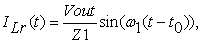(9)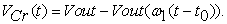(10)

As well as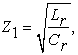(11)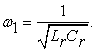(12)

The initial amount of Cr capacitor voltage and Lr inductor current is zero. Due to the fact that the current can appear in Cout output capacitor, through Saux2 after turning off Saux1 as well as for the sudden decrease, increase of current in Cout that would cause the output spike voltage from Ls inductor that is used with "spike-killer" term which is placed in series with Saux2.

3.1.3. The Third Condition of (C) Figure

In this mode, a situation that is t1<t<t2 and t = t1, with the discharge of Cout capacitor, that will be done by changing the polarity of the capacitor, that at this moment no current will flow through diode D4 and it will act as an open switch in the circuit, discharging Cr capacitor and Lr inductor will cause charging of Cs capacitor and the auxiliary switch current is the total input currents to negative polarity of voltage main supply and Cs capacitor current. The Cr capacitor voltage equation of Lr current is calculated in this mode: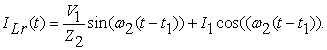(13)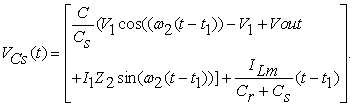(14)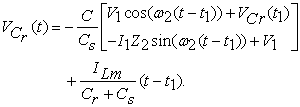(15)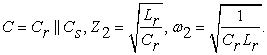In this condition the Lr inductor current will reach its maximum value that is Vcs − Vcr = 0 that is equal to the maximum value of auxiliary calculated switch current: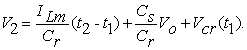(16)

3.1.4. The Fourth Condition of (D) Figure

In this mode, the condition that is t2<t0<t3 and t = t2 the Cs capacitor has been fully discharged and current transmits through body diode of main switch; in this mode the switches can be turned on through ZVS. The Cr capacitor voltage equation of Lr current is calculated in this mode: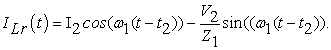(17)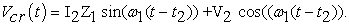(18)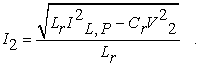(19)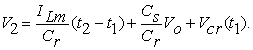(20)

3.1.5. The Fifth Condition of (E) Figure

Under this mode, that is a t3<t<t4 and t = t3 condition, this condition is similar to the fourth condition and the current transmits through body diode of main switch that all relations in this mode are similar to fourth condition.

3.1.6. The Sixth Condition of (F) Figure

In this mode, that is t4<t<t5, no current will transmit through auxiliary switches and in t = t4 the Cr capacitor and Lr inductor will be resonance. In this mode Saux1 auxiliary switch can be turned off by ZCS. ZCS circuit is shown in Figure 11:

• Figure 12. Current waveform and ZVS voltage on IGBT bases

When Saux1 switch is on and before the current increases, the drain base voltage almost becomes zero and as a result of losses we will have lower on-state on the switch. In addition, the stored energy in capacitor will be lost between the source and drain stands. On the other hand this is worth mentioning that this capacitor is of high importance in higher frequency. In ZCS topology when the switch is turned off, the voltage across the drain and source will be close to zero and reversed (i.e. current will flow through parallel diode to switch) that can prevent the current direction change (reversing) in the resonant circuit. Switch is cut off (Figure 12) during the reversal of flow. So, almost no losses are recorded during the shutdown 21.

3.1.7. The Seventh Condition of (G) Figure

In this mode, that is the situation t5<t<t6 and in t = t5, the Saux2 auxiliary switch is turned on and the Cr capacitor is discharged through Lr inductor, the Cr and Lr current will be zero and Saux2 auxiliary switch can be turned off by ZCS. Cr capacitor voltage equation of Lr current has been calculated in this mode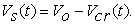(21)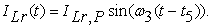(22)

3.1.8. The Eighth Condition of (H) Figure

In this mode, that is the situation t6<t<t7, Cout output capacitor is discharged and the current flow is closed through the parallel capacitor with Saux1 auxiliary switch, In addition, the voltage drop across the capacitor is much smaller than the capacitor Cr. In the following, the equation related to Saux1 auxiliary switch voltage has been calculated: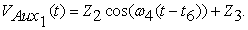(23)

Where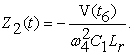(24)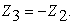(25)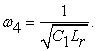(26)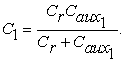(27)

It should be noted that the Saux1 connected capacitor has been much less charged than the output capacitor charged.

3.1.9. The Ninth Situation of (I) Figure

In this mode or the condition that is t7<t<t8, the main switch is turned on and in the meantime the input inductor current is in dropping state.

3.1.10. The Tenth Condition of (J) Figure

In this mode or the condition that is t8<t<t9 in t = t8, under such condition all switches are turned off and Cs capacitor voltage is calculated through following equation:(28)

As a result, the capacitor voltage of Cr auxiliary circuit and Cs capacitor are discharged and parallel diode is reversed, the energy stored in the Cr capacitor is transmitted to the output.

• Figure 13. Current waveform before applying active filter
• Figure 14. Dc capacitor voltage waveform in PWM block

As shown in Figure 15, after starting the parallel active filter of supply currents become similar to the phase voltages that means the parallel active filter compensates very well the reactive power. Strokes exist in supply current specified in Figure 17 these strokes occur due to high di/dt in load currents and they cannot be fully compensated or removed by parallel active filter. To remove the stroke existing in the source current, greater di/dt should be permitted in ica, icb, icc compensatory currents. One way to do this is to decrease the inductor of L commutation reactors.

• Figure 15. Source current waveform after applying the active filter
• Figure 16. Dc capacitor voltage waveform of Soft Switch output on the PWM block
• Figure 17. Source current waveform after applying active filter

### Acknowledgments

In this paper, a new topology has been employed using the soft switching to improve the performance of parallel active filter (SAPF). The proposed inverter can be used under ZVS zero voltage switching conditions with high frequency without using Snubber capacitor. The soft switching effectively improves the performance of active filter and lowers the stress voltage level and the switch voltage strokes and will have reduced EMI dissemination. In addition, the new topology will eliminate the problem of reverse recovery of body diode components and in addition the voltage stress on the PI controller, will greatly reduce used in the power active filter. The laboratory model of improved parallel active power filter has been designed by Soft Switch in the range of 2.5 KVA, and the experimental results showed the high performance of soft power switch on the performance of active power filter.

### References

  J. S.Tepper; J. W. Dixon; G. Venegas; L. Moran IEEE Transactions on Industrial Electronics, A simple frequency-independent method for calculating the reactive and harmonic current in a nonlinear load, Year: 1996, Volume: 43. In article  B. Singh, K. Al-Haddad, A. Chandra, "A review of active filters for power quality improvement", IEEE Trans. On Industrial Electronics, Vol. 46, No. 5, pp. 960-971, Oct. 1999. In article View Article  A. Massoud, S.J. Finney, B.W. Williams, "Review of harmonic current extraction techniques for an active power filter", Proceeding of the IEEE/ICHQP, pp. 154-159, Sep. 2004. In article View Article  V. Pires, J. Silva, "A current source active power filter controlled by a sliding mode approach", Proceeding of the IEEE/EPEPEMC, Vol. 1, pp, 1654-1659, Portoroz, Aug. 2006. In article  K. G. Firouzjaz, A. Sheikholeslami, M. R. Karami and F.Heydari, “Predictive Current Control Method for Shunt Active Filter with Windowing Based Wavelet Transform in Harmonic Detection,” Simulation Modelling Practice and Theory, Vol. 17, No. 1, 2009, pp. 883-896. In article View Article  N. Mendalek, K. Al-Haddad, H.Y. Kanaan, G.Hassoun, "Sliding mode control of three-phase four-leg shunt active power filter", Proceeding of the IEEE/PESC, Vol. 1, pp. 4362-4367, June. 2008. In article View Article  W.R. Nogueira Santos, E.R. Cabral da Silva, C. Brandao Jacobina, E. de Moura Fernandes, A. Cunha Oliveira, R. Rocha Matias, D. Franca Guedes Filho, O.M. Almeida, P. Marinho Santos, "The transformerless single-phase universal active power filter for harmonic and reactive power compensation", IEEE Trans. on Power Electronics, Vol. 29, No. 7, pp. 3563-3572, July 2014. In article View Article  B. Singh, K. Al-Haddad," A review of active filters for power quality improvemen" IEEE trans on Industrial Electronics, Vol. 46, NO 5, October 1999. In article View Article  N.VANAJAKSHI, G.NAGESWARA RAO, "A THREE PHASE SHUNT ACTIVE POWER FILTER FOR HARMONICS REDUCTION", IRJET, V. 02 Issue: 09, Dec-2015. In article  L. S. Yang, and T. J, Liang, “Analysis and Implementation of a Novel Bidirectional DC-DC Converter,” IEEE Trans. Industrial Electronics, vol. 59, no. 1, pp. 422-434, Jan. 2012. In article View Article  M. R. Mohammadi and H. Farzanehfard, “New Family of Zero- Voltage-Transition PWM Bidirectional Converters With Coupled Inductors,” IEEE Trans. Ind. Electron., vol.. 59, no. 2, pp. 912-919, Feb. 2012. In article View Article  S.B. Yaakov,“Average Simulation of PWM Converters by Direct Implementation of Behavioral Relationships” IEEE Applied Power Electronics Conference, 1993. In article  P. Das, B. Laan, S. A. Mousavi, and G. Moschopoulos, “A nonisolated bidirectional ZVS-PWM active clamped DC-DC converter,” IEEE Trans. Power Electron., vol. 24, no. 2, pp. 553-558, Feb. 2009. Nonisolated Bidirectional ZVS-PWM Active Clamped DC-DC Converter,” IEEE Trans. Power Electron., vol. 24, no. 2, pp. 553- 558, Feb. 2009. In article View Article  W. Chen, P. Rong,,, Z. Lu, “Snubberless Bidirectional DC-DC Converter With New CLLC Resonant Tank Featuring Electronics, vol. 57, no 9, pp. 3075-3086, 2010. In article  And G. Marques, "A control method for active power filters under unbalanced non-sinusoidal conditions," in PEVD'96 september, 23-25, pp. 120-124. In article  H.Akagi, Y.Kanazawa andA.Nabae,"Instantaneous reactive power compensator comprising switching devices without energy storage components," IEEE Trans. Ind. Appl. vol. IA-20, 1984, pp. 625-630. In article View Article  H.Akagi, Y.Kanazawa, A.Nabae,” Generalized theory of the instantaneous reactive power in three-phase circuits,” IPEC’83-Int. Power Electronics Conf.,Tokyo, Japan, 1983, pp.1375-1386. In article  Pankaj H Zope, Pravin G.Bhangale, Prashant Sonare, S. R.Suralkar. "Design and Implementation of carrier based Sinusoidal PWM Inverte. "International Journal of Advanced Research in Electrical, Electronics and Instrumentation Engineering, Vol. 1, Issue 4, October. In article  Da Silva, E.R.C. Cavalcanti, M.C.; Jacobina, C.B. Comparative study of pulsed DC-link voltage Converters. IEEE Trans. Power Electron. 2003, 18, 1028-1033. In article View Article  Pulse-width Modulated DC-DC Power Converters MARIAN K. KAZIMIERCZUK Wright State University Dayton, Ohio, USA. ISBN: 978-1-119-00954-2. In article  Pulse-width Modulated DC–DC Power Converters MARIAN K. KAZIMIERCZUK Wright State University Dayton, Ohio, USA. In articleThis work is licensed under a Creative Commons Attribution 4.0 International License. To view a copy of this license, visit http://creativecommons.org/licenses/by/4.0/